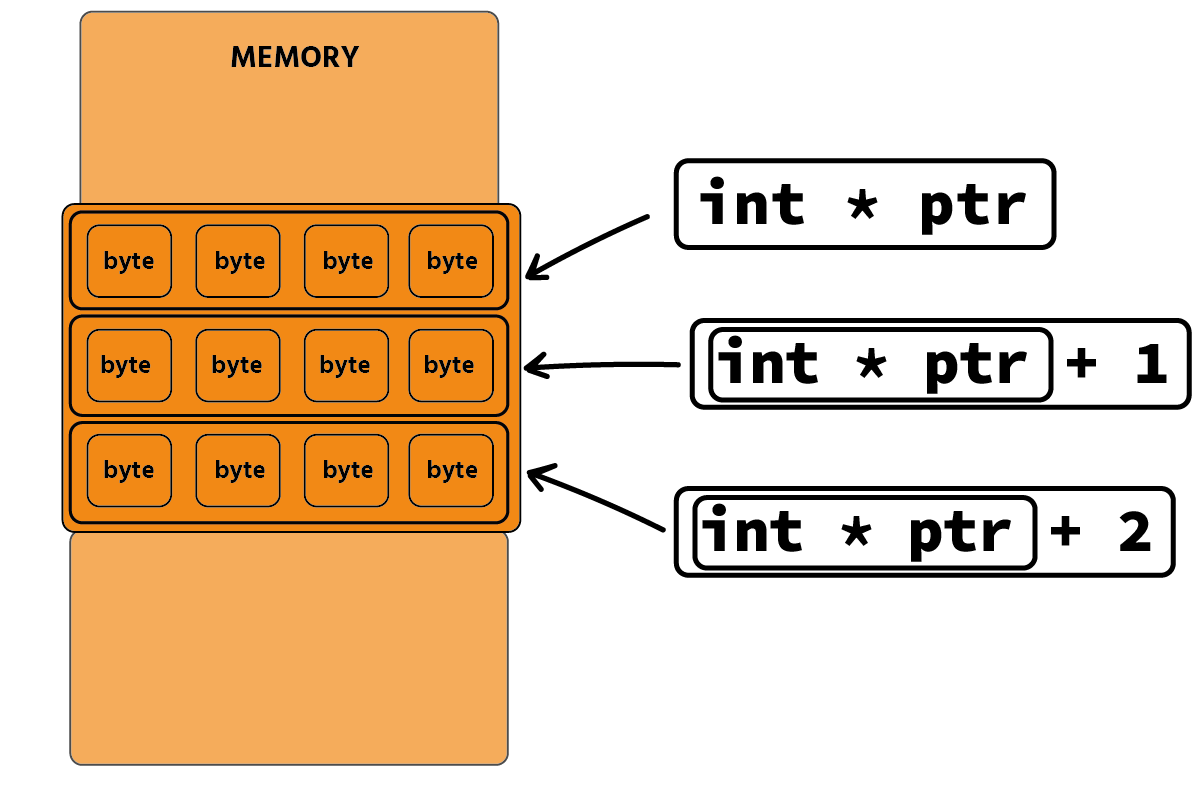Course Content

Introduction to C++Address Arithmetic (Bonus)

The referencing/dereferencing operation is not the only operation that can be performed on addresses. Arithmetic operations can be applied to addresses.

For example, let's increase the address by 1:main.cppNote

Imagine that you walk 4 meters in 1 step. If you add 1 step to your position, you will move 4 meters.

When we add 1 to an address, we increment the address by the value of its data type in bytes. An int pointer points to a cell of 4 bytes because an int takes 4 bytes. Therefore, after increasing the address by 1, we will move forward with 4 bytes. This works with all data types. The shift will occur exactly as many bytes as the data type of the pointer is specified.main.cppFrom this point of view, you can look "under the hood" at arrays.

## Arrays don't exist?

An array is a collection of pre-allocated memory locations that we can navigate through using address arithmetic. The address of an array always points to its beginning.main.cppDereferencing an array returns the first element of the array. If we add the number 1 to the array and apply the dereference operator to the result, we get the following array element:main.cppTo display the entire array, you can use a for loop:main.cppAs you can see, the address increases by 4 bytes in each iteration.

Section 6.

Chapter 5